## Introduction

Algebra is a branch of mathematics which uses letters or symbols to represent unknown quantities. It includes the rules to manipulate these symbols. The use of letters helps us to form equations relating to the unknown and known quantities, and generate formulae to calculate areas, perimeters, volumes, bank interests, etc.

Maths is not all about numbers; sometimes, we are presented with a problem where it is not possible to know all values, and we must use variables to represent these unknown values. Algebra teaches you how to deal with these variables in equations.

Let us watch this video to understand the need for algebra:

We saw that there are many situations where there is some quantity whose value we do not know. We can represent the relation between the known and unknown quantities using algebra. Such quantities are represented using any letter of the English alphabet. Example: x, y, p, q, a, b, c, etc.

Can you think of a real-life example where you use algebra?

Suppose you have school at 8 AM every day. What is the first thing you do in preparation the night before? You will probably set an alarm by calculating the approximate time you need to wake up, brush, shower, and have breakfast before heading out. You will also factor in the time required to reach from your house to your school and set your alarm accordingly. Unknowingly here, you have used algebra to set your morning routine! Since you did not know exactly how much time each of your morning activity requires, you have used the concept of variables to get an approximation.#### Concepts

The chapter ‘Algebra’ covers the following concepts:

#### Variables and Constants

In some situations, there can be quantities whose value can vary. Such quantities are represented using any letter of the English alphabet and are called variables. Example: x, y, p, q, a, b, c etc. This branch of Mathematics which deals with numbers and variables is called Algebra. Real life examples of variables include: the number of people visiting a library on different days, runs scored by a player in different matches, etc.

There are certain quantities which do not change their value; they remain fixed. Such fixed values are called constants. Example, numbers such as 4, 26, 100, etc. are constants.

• If a term has a variable, then it is called an ‘Algebraic term’.
• If the term is just a number, then it is called a ‘Constant term’.#### Algebraic Expressions

Algebraic terms are terms with variables. They can take different values depending on the value of the variable. Constant terms are just numbers which have a fixed value. Terms combined with mathematical operators make an algebraic expression.

An algebraic expression is a mathematical statement containing variables. An algebraic expression can be described as a combination of constants and variables with four mathematical operations, namely addition, subtraction, multiplication and/or division.

Consider the following statement:This can be written in a mathematical statement as:Let us see a few more such statements.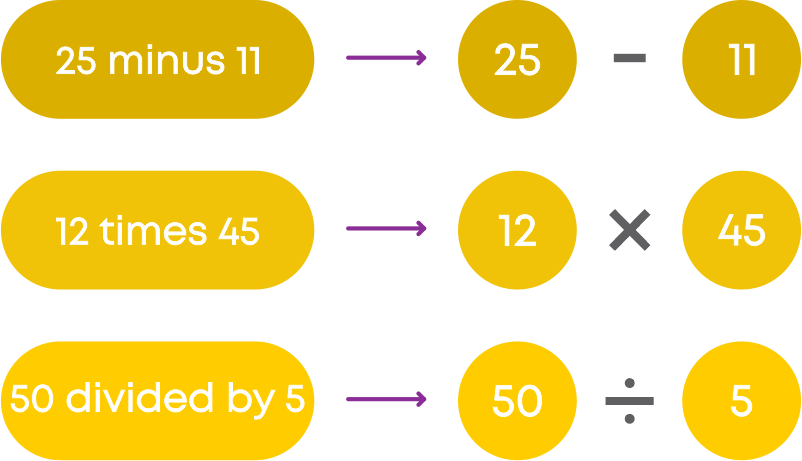Remember, numerical values in a statement are always fixed and constant.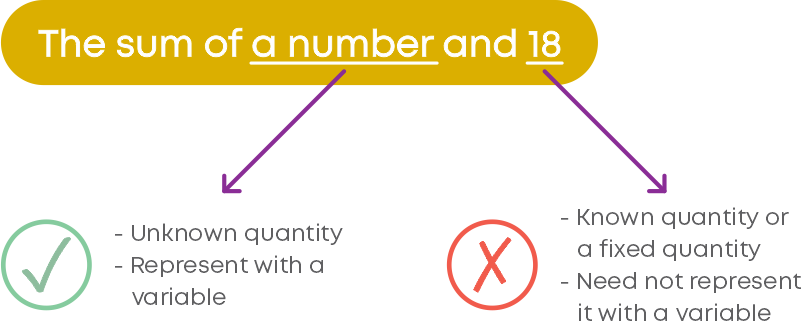Now let us learn how to form rules for different number patterns. Consider this pattern:Let us make an observation.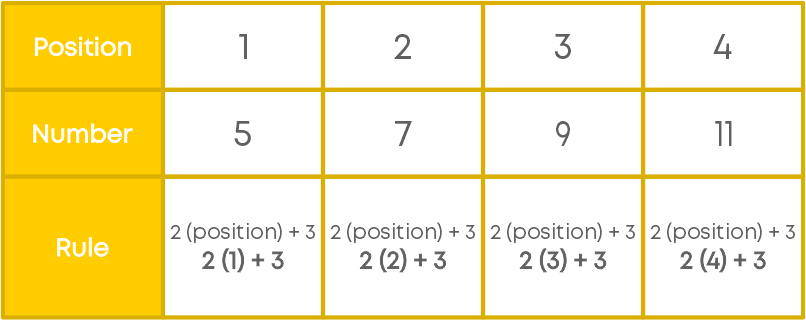If we consider the position of each number as ‘m’, then the rule will be:So, we can form a rule for number patterns also. To form a rule for any given geometrical or number pattern:

• Identify the relation between two consecutive shapes or numbers.
• Form a relation between the position and the shape or number.
• Represent the changing value using a variable.
• Write the rule using the variable.

How do you write the correct rule matchstick patterns? Count the number of matchsticks in each shape of the pattern. Observe the position of the shape also.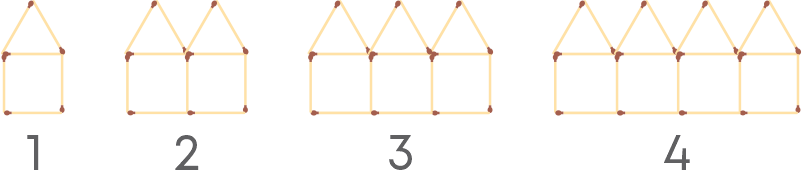Now, identify the pattern and its relationship with the position. Here, we see that in each case the number of matchsticks used is:

5 × position number + 1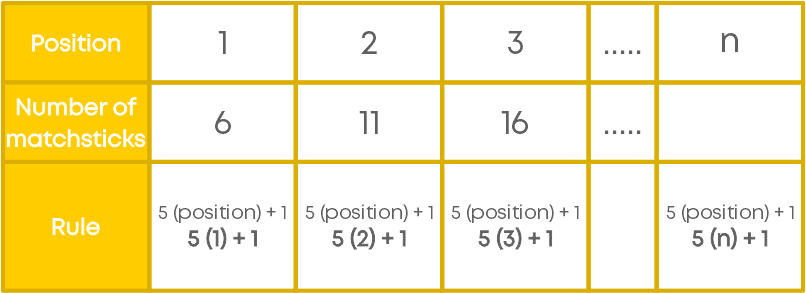Here, the position number of the shape is varying, hence, we represent it with a variable ‘n.’ The rule will be 5n + 1.

We can form algebraic expressions for given verbal statements involving different operations. Let us see how:

While converting a statement into an algebraic expression:

• Identify the unknown quantity in the statement.
• Identify the operation needed to relate the known and unknown quantities.
• ‘added’ or ‘sum of’ or ‘increased by’ or ‘more than’ – All mean addition.

Subtraction

When a statement is converted into an algebraic expression:

Identify the

unknown quantity.

Identify the operation needed to relate the known and unknown quantities.

‘Minus,’ ‘difference,’ ‘decreased by,’ ‘less than’ – All mean subtraction.

Multiplication

When a statement is converted into an algebraic expression:

• Identify the unknown quantity in the statement.
• Identify the operation needed to relate the known and unknown quantities.
• ‘Multiplied by,’ ‘times,’ ‘of’ – All mean multiplication.
• Sometimes you may have more than one operation involved.

Division

When a statement is converted into an algebraic expression:

• Identify the unknown quantity in the statement.
• Identify the operation needed to relate the known and unknown quantities.
• ‘Quotient,’ ‘divided by’ – All mean division.
• Sometimes you may have more than one operation involved.

Mathematical rules and formulae can be written as algebraic expressions. For example, the area of a rectangle is calculated as the product of the length and the breadth of the rectangle, i.e., area = length × breadth. We can write this formula as an algebraic expression by considering the length and the breadth of a rectangle as variables, namely ‘l’ and ‘b,’ respectively.

So, the formula to find the area of a rectangle can be written as:

Area = l × b = lb

For any shape such as a square, rectangle, triangle or circle, the perimeter is the distance around the shape. The perimeter can be written in algebraic form as the sum of all sides.

Algebraic expressions help us represent any rule as a general expression.

Now let us learn how to form an algebraic expression for commutative property of whole numbers. We know that when we add two whole numbers, the order in which we add them does not matter. In general, we can represent two whole numbers as ‘a’ and ‘b’.

Hence, according to the commutative property of addition for whole numbers, we haveLet us recall another important property of whole numbers. While multiplying three or more whole numbers, we can group the numbers and multiply. How we group the numbers does not really matter. So, in general, for any three whole numbers ‘a’, ‘b’ and ‘c’, we can state the associative property of multiplication as: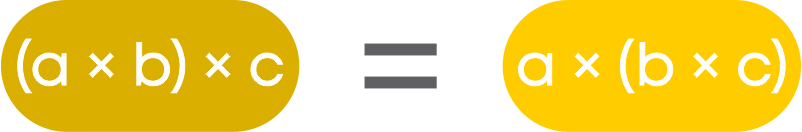Similarly, we can represent the distributive property of whole numbers (‘a’, ‘b’ and ‘c’) as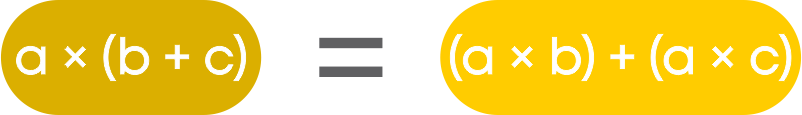#### Algebraic Equations

An algebraic equation has an ‘=’ sign with expressions or constants on either side of it. For e.g., 5x + 9 = 19. In this equation, we see that variables are also involved. In other words, algebraic terms are involved.

• The expression on the left of the ‘=’ is called the Left-Hand Side or LHS.
• The expression on the right of the ‘=’ is called Right-Hand Side or RHS.

An algebraic expression is just a combination of algebraic terms. There is no ‘=’ sign in it. For e.g., 4x + 6.

Can we form equations for a given mathematical statement in verbal form? To form an equation from a statement, we follow the steps given below:

• Identify the unknown and represent it with a variable.
• Identify the operation to be used.
• Look out for terms such as ‘added to’, ‘sum of’, more than’ or ‘increased’. These terms indicate that the operation to be used is addition (+).
• Form the equation.

What does the ‘equal to’ sign indicate in an equation? The equal sign indicates that both sides of the equation are equal, i.e., the equation is true only if the left- hand side expression is equal to the right-hand side expression.

Let us understand the meaning of satisfying the equation or solution of an equation. For example, consider the linear equation 5x + 12 = 27 where x = 3.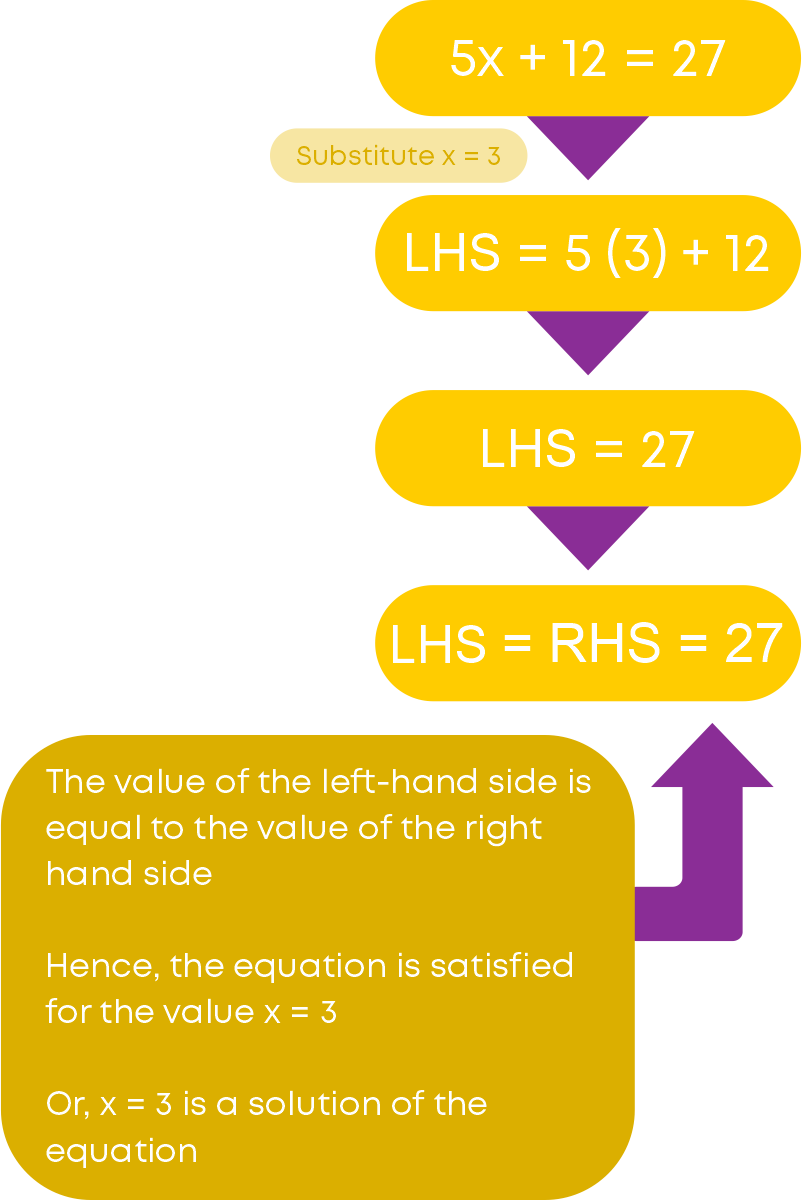Let us take the value of ‘x’ to be 2.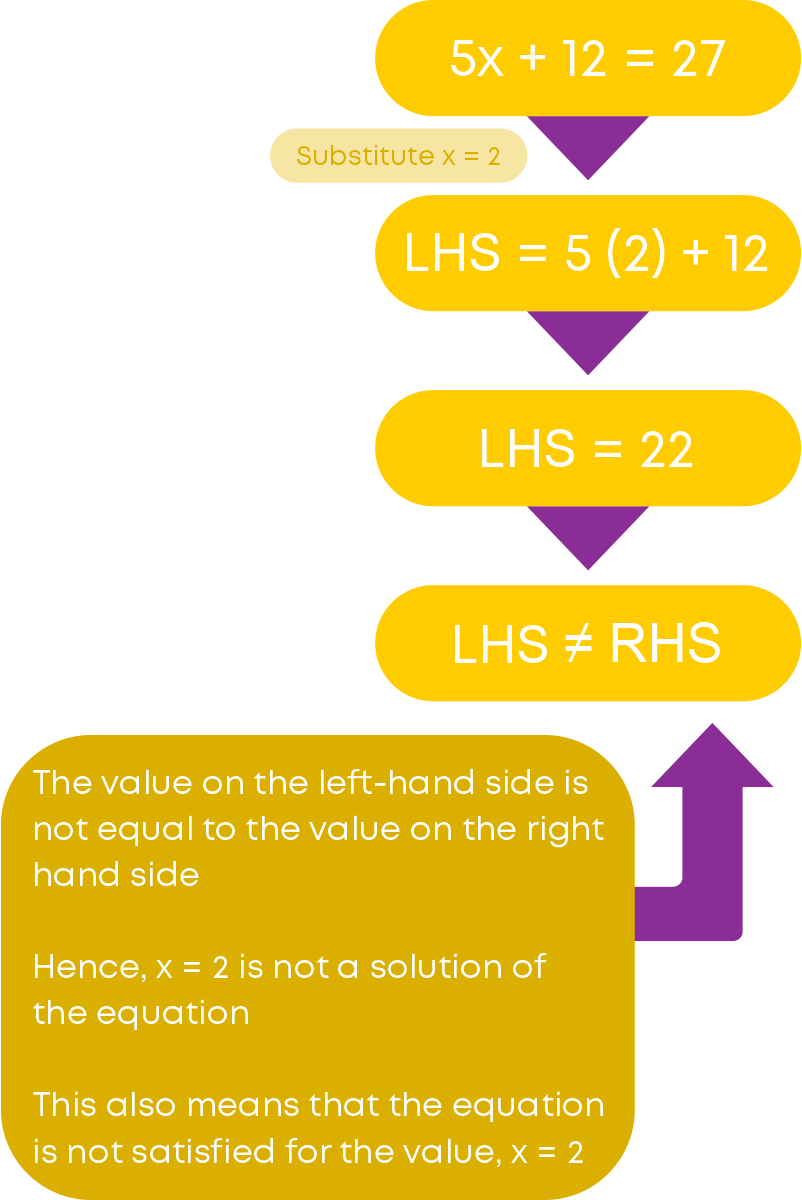So, we can conclude that x = 2 is not a solution to the equation 5x + 12 = 27.

This method of substituting different values and arriving at the value which satisfies the equation is called the Trial and Error method.

To check if a given value is a solution of an equation: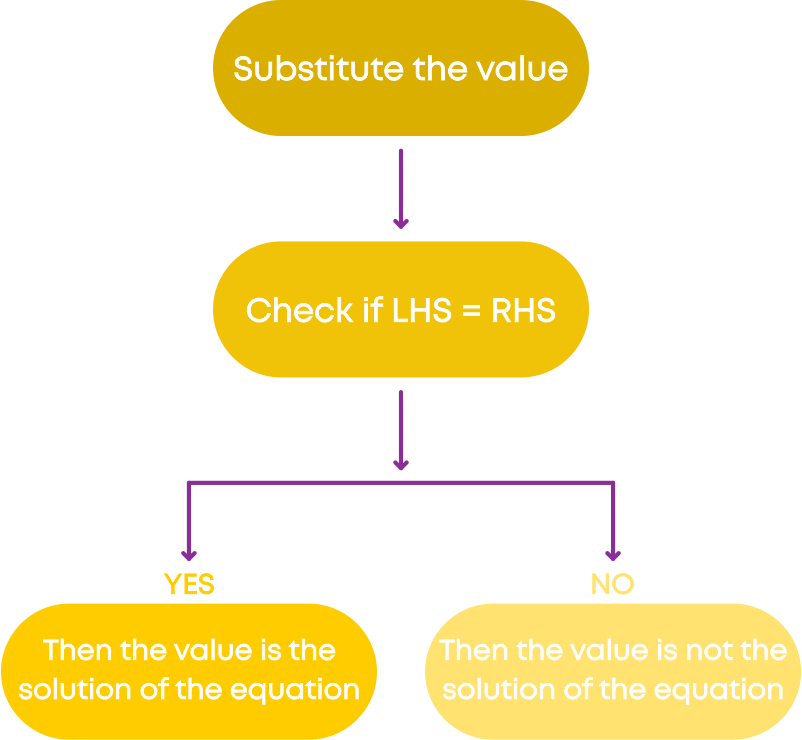While solving real-life problems, we follow this strategy:

• Analyse the question to identify the known and unknown quantities.
• Form an equation relating the quantities.
• Solve the equation to find the value of the unknown.

#### Common Errors

The following are the topics in which students make common mistakes when dealing with algebra

• 1. Identifying the unknown quantity
• 2. Writing a rule for matchstick patterns
• 3. Forming an algebraic expression for a given statement
• 4. Check whether the given value is a solution of the equation

#### Identifying The Unknown Quantity

In a given situation, we can have quantities which are known and quantities which are not known. We need to identify the unknown quantity correctly and represent it with a letter or variable. Example, consider the statement:How can we express this statement in mathematical form? Here, we do not know what is the number. Hence, it is an unknown quantity. We represent this with a variable, let us say ‘x’. The number 18 is a known quantity.

Remember, numerical values in a statement are always fixed and constant. The statement above can be represented mathematically as ‘x + 18.’#### Writing A Rule For Matchstick Patterns

How to write the correct rule to form the given pattern of matchsticks? Count the number of matchsticks in each shape of the pattern. Observe the position of the shape also.Now, identify the pattern and its relationship with the position. Here, we see that in each case the number of matchsticks used is:

5 × position number + 1Here, the position number of the shape is varying, hence, we represent it with a variable ‘n.’ The rule will be 5n + 1.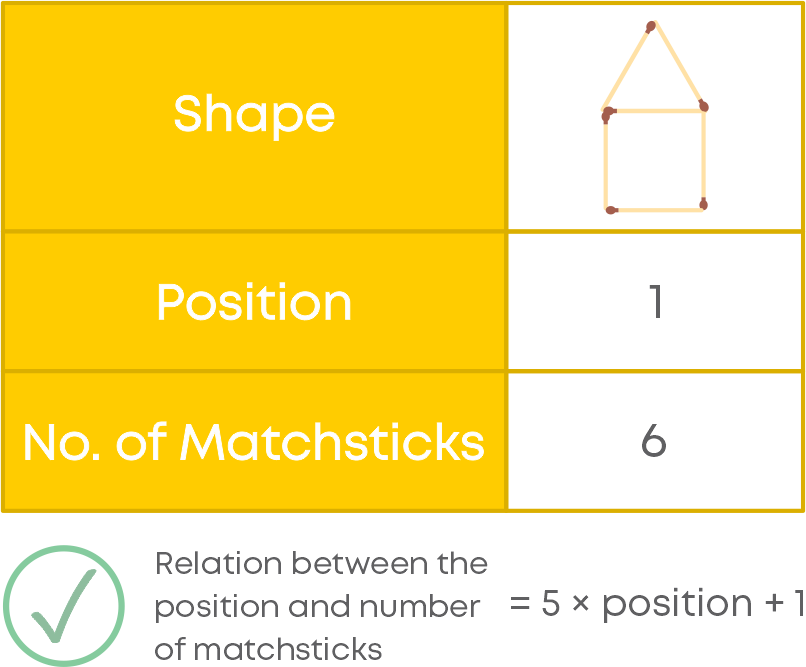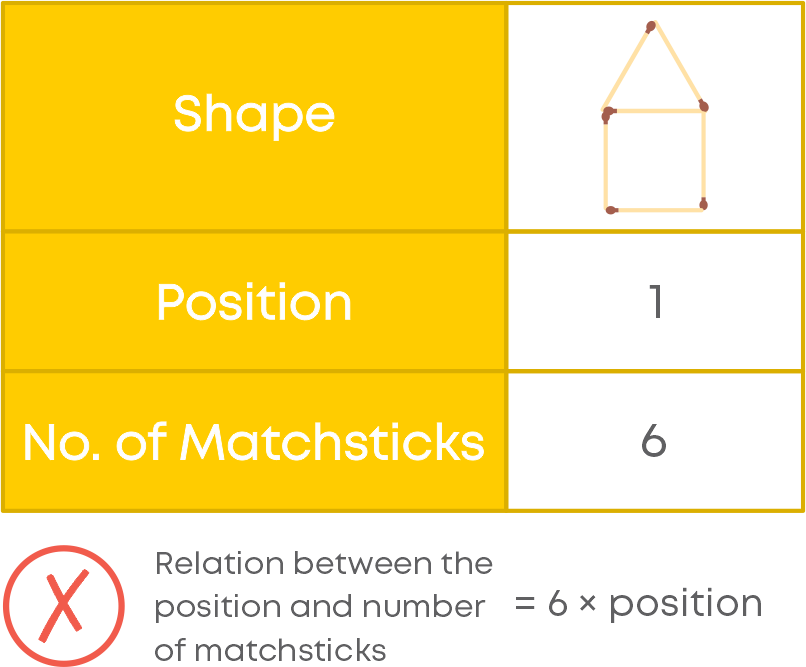#### Forming An Algebraic Expression For A Given Statement

To form an algebraic expression from a given statement, we must:

• Identify the unknown quantity and represent it with a variable
• Identify the constant or fixed quantity
• Identify the operation which relate the known and unknown quantities

Take care of the operation. Example, consider the statement:Twice a number means 2 multiplied with the number. Added means addition or '+.' So, the correct statement will be 2p + 12.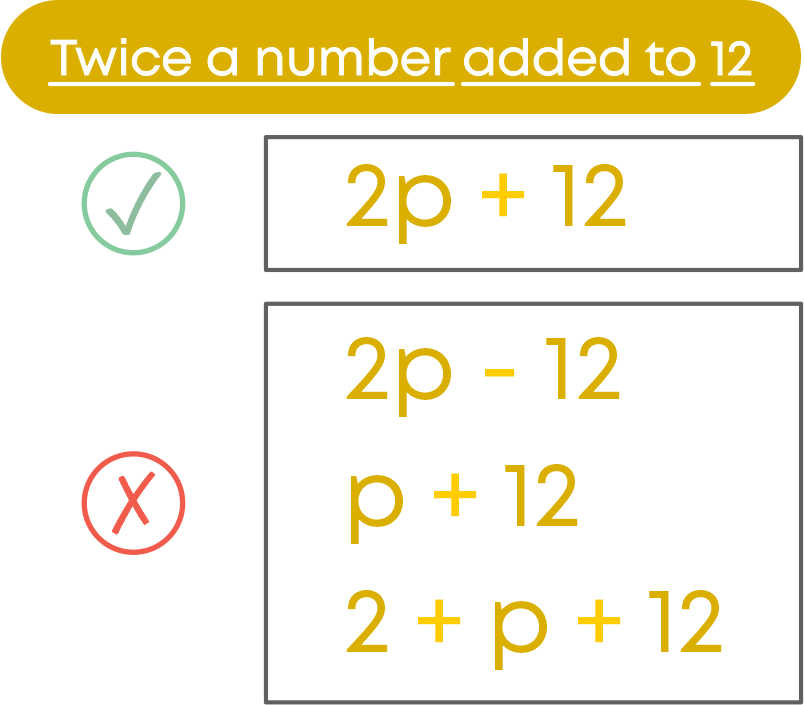#### Check Whether The Given Value Is A Solution Of The Equation

To check whether a given value is a solution of the equation:

• Observe the equation carefully for the variable
• We must put the value in place of the variable
• Perform the operations carefully with the numbers

Example, check if 4 is the solution of the equation:Observe that both LHS and RHS have the variable ‘y’. Put the value of ‘y’ in both the sides and find the value.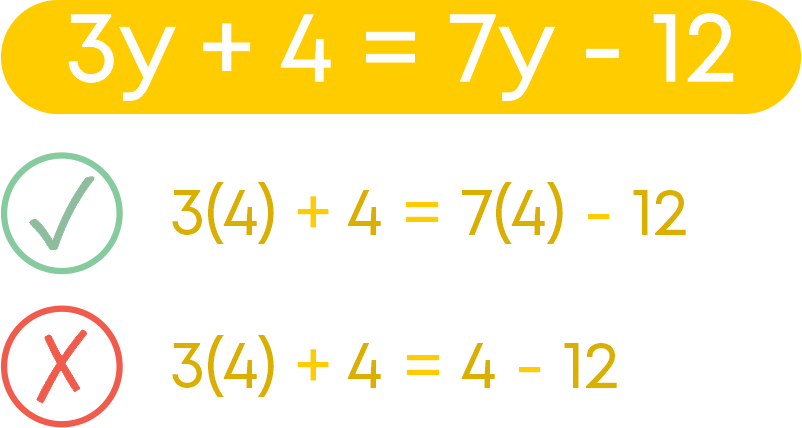#### Conclusion

Algebra is an interesting chapter with many applications in real life. Now that you have learnt all about it, can you solve the puzzle below?

Find the value of each colour of the balloons below: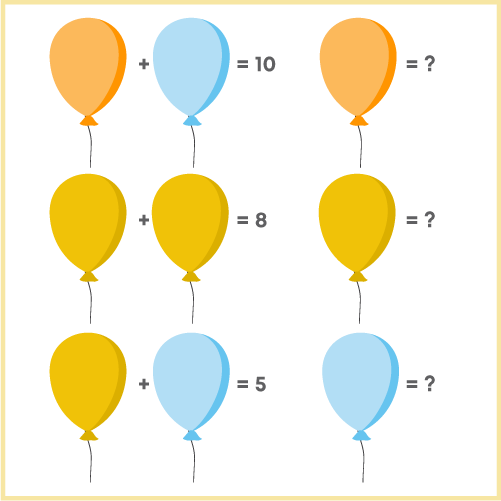#### Arpana

Author

Arpana is an education specialist with years of teaching Math and developing content. She previously worked as a freelance content developer, developing lesson plans for a reputed publisher of text books and content for various educational companies. She is an enthusiastic teacher who has taught students from India, the UK, and New Zealand.